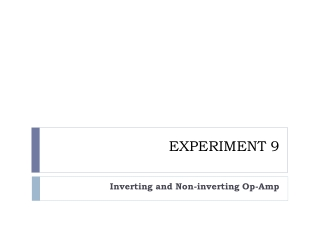DownloadDownload PresentationEXPERIMENT 9

# EXPERIMENT 9

Télécharger la présentation## EXPERIMENT 9

- - - - - - - - - - - - - - - - - - - - - - - - - - - E N D - - - - - - - - - - - - - - - - - - - - - - - - - - -
##### Presentation Transcript

1. EXPERIMENT 9 Inverting and Non-inverting Op-Amp

2. PART A INVERTING AMPLIFIER

3. LM741

4. Set up the oscilloscope to show a dual trace, one for the input of the op-amp and one for the output. Use DC input coupling. Set up the function generator to produce a sine wave with an amplitude of 1 V peak-to-peak and frequency 2 kHz. Make sure that the grounds of the function generator, oscilloscope, and prototyping board are somehow connected.

5. Measure the values of the resistors, then using the breadboard, set up the inverting amplifier shown in Fig. 9.1 where R1 = 10 k and RF = 100 k

6. GND GND -15 V +15 V

7. Measure the voltage between pin 2 and ground. This is known as virtual ground. Record the voltage and comment on your observation. • From the output waveform, deduce the gain of the amplifier and verify that the amplifier inverts. • Next, repeat step 4 by replacing R1 with the 30 kand 20 kresistors • Using the measured values of the resistors, calculate the ratio of RF/R1 and compare whether the magnitudes are equal to the magnitude of the gain that have been deduced from step 4.

8. Part A (Inverting Amplifier) : a) R1k(measured ) = _______________ b) R10k ( measured ) = ________________ c) R20k ( measured ) = ________________d) R30k ( measured ) = ________________ e) R100k ( measured ) = ________________ Voltage measurement between pin 2 and ground = _________________ Gain of the amplifier = vo (peak) / vi (peak) = _______________ a) When R1 = 30 k : Gain of the amplifier = vo (peak) / vi (peak) = ______________ b) When R1 = 20 k : Gain of the amplifier = vo (peak) / vi (peak) = ______________ 0 V -10 -3.33 -5

9. Ratio of RF /R1 R1 = 10 k : RF /R1 = ______________________ R1 = 30 k : RF /R1 = _______________________ R1 = 20 k : RF /R1 = _______________________ Comment of the comparison between the magnitudes: 10 3.33 5

10. Using R1 as 20 k , now place a load resistor, RL = 1 k between pin 6 and the ground. • Repeat step 4. Comment on your observation. Is there any change in the value of the gain? - 5 • With load resistor, RL = 1 k, gain of the amplifier = ________________ • Comment:

11. GAIN = -10 GAIN = -3.33

12. GAIN = -5